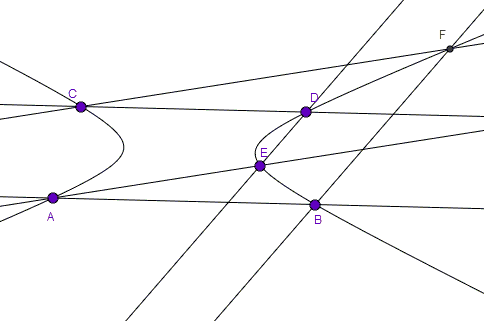# Three pairs of Parallel Lines and a Conic

### Problem

Six points A,B,C,D,E,F are such that AB||CD, AE||CF, and BF||DE.Prove all six points lie on a conic.

### Hint

If the statement is correct, and there is indeed a conic through the six points, you can change the configuration to a more convenient one by an affine transformation without disturbing its essential properties: keeping parallel lines parallel, conic passing through the six points.

### Solution

There is a conic through five points A,B,C,D,E. Below, this is the conic I shall refer to.

First apply a sheer transform parallel to and fixing AB that makes AC and BD mirror symmetric in the perpendicular bisector of AB:This I believe (but need to have proved) makes also the conic symmetric in the perpendicular bisector.

Next, squeeze or stretch the diagram in the perpendicular direction to get a circle.In this configuration, point E also lies on the circle. This is because the arcs BAC and ABD are equal as are the angles BFC and AED. Since the latter is inscribed into the circle, so is the former.

The above construction may have a virtue of helping one's intuition; unfortunately it contains major gaps. Not least of which is that it may not work when the conic is not an ellipse, like in the diagram below:Which suggests that the statement is of a projective rather than affine character so that the given lines need not be pairwise parallel. Likely, what is needed as that the lines belong to three collinear pencils of lines:Why does it remind me of Pascal's theorem? This is because the hexagon ABFCDE appears to produce Pascal's line which moves to infinity when the pencils comprise parallel lines.

### Acknowledgment

The problem has been posted by Dao Thanh Oai at the CutTheKnotMath facebook page.